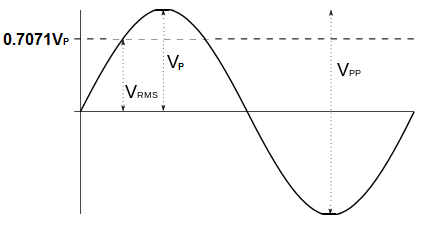﻿ RMS Voltage Calculator ﻿# RMS Voltage CalculatorV

0.0000V

V

0.0000V

### Average Voltage to RMS Voltage Calculator

V

0.0000V

The RMS Voltage calculator calculates the RMS Voltage value from either the peak voltage, the peak-to-peak voltage, or the average voltage. It calculates the RMS Voltage based on the above formulas for each respectively.

To compute the RMS voltage from the peak voltage, the peak voltage is multiplied by 0.7071.

To compute the RMS voltage from the peak-to-peak voltage, the peak-to-peak voltage is multiplied by 0.35355.

To compute the RMS voltage from the average voltage, the average voltage is multiplied by 1.1107.

A user enters the voltage, V, and the result will automatically be calculated and shown. The RMS voltage result which is displayed above is in unit volts (V).

RMS voltage is a method of representing an AC voltage waveform in which the RMS value specifies the amount of DC voltage that will produce the same heating effect, or power dissipation, in a circuit, that the AC signal would over its entire cycle.

Thus, the RMS value is a very important value when comparing AC signals to DC signals, in that they both produce the same amount of heating in a circuit and cause the same amount of dissipation of power.

Related Resources

﻿